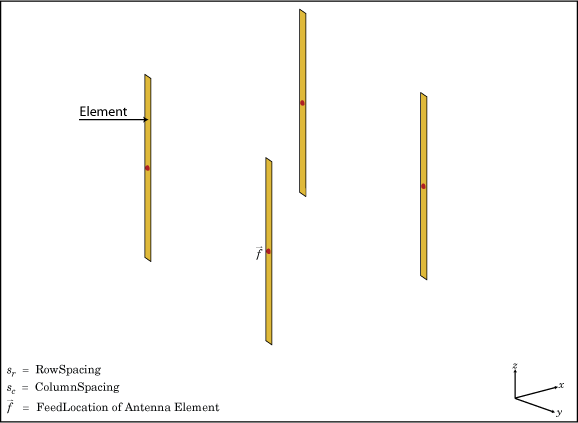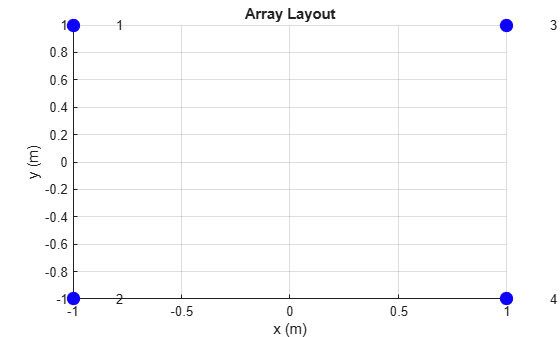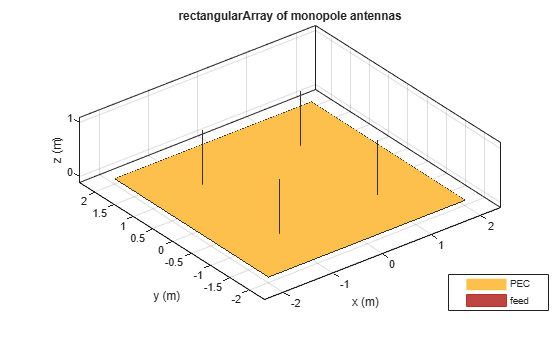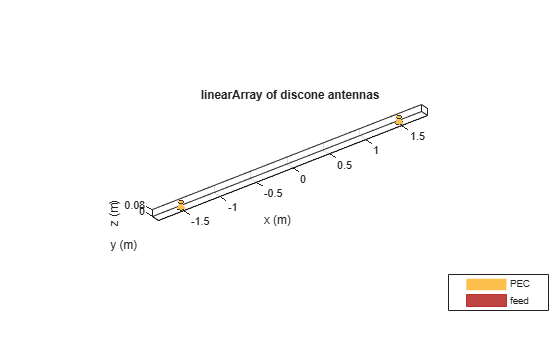# rectangularArray

Create rectangular antenna array

## Description

The `rectangularArray` class creates a rectangular antenna array in the xy-plane. The default rectangular array is a four-element dipole array in a 2-by-2 rectangular lattice. The dipoles are center-fed. Each dipole resonates at 70MHz when isolated.## Creation

### Syntax

``array = rectangularArray``
``array = rectangularArray(Name=Value)``

### Description

example

``` `array = rectangularArray` creates a rectangular antenna array in the xy-plane.```

example

``` `array = rectangularArray(Name=Value)` creates a rectangular array, with additional Properties specified by one or more name-value arguments. `Name` is the property name and `Value` is the corresponding value. You can specify several name-value arguments in any order as `Name1=Value1`, `...`, `NameN=ValueN`. Properties that you do not specify retain their default values.```

### Output Arguments

expand all

Rectangular array, returned as an `rectangularArray` object.

## Properties

expand all

Individual antenna type, specified as a single antenna object for homogeneous array or a vector of antenna objects of same type with varying dimensions and load for heterogeneous array. The antenna object can be an element from the Antenna Catalog, or a `linearArray` or `rectangularArray`, or an antenna without a substrate created using `customAntennaGeometry`, `customAntennaMesh`, `customAntennaStl`, or `customAntenna` objects.

Note

Following antennas are unsupported as an element:

Example: `monopole`

Example: `[monopole monopole(Height=2)]`

Example: `rectangularArray`

Example: `customAntenna`

Number of antenna elements in row and column of array, specified as a two-element vector.

Example: `[4 4]`

Row spacing between two antenna elements, specified as a scalar or vector in meters. By default, the antenna elements are spaced 2m apart.

Example: `[5 6]`

Data Types: `double`

Column spacing between two antenna elements, specified as a scalar or vector in meters. By default, the antenna elements are spaced 2m apart.

Example: `[3 4]`

Data Types: `double`

Antenna elements spatial arrangement, specified as a text input.

Example: `"Triangular"`

Data Types: `string`

Excitation amplitude of antenna elements, specified as a scalar or vector. Set the property value to `0 ` to model dead elements.

Example: `3`

Data Types: `double`

Phase shift for antenna elements, specified as a scalar or vector in degrees.

Example: `[3 3 0 0]`

Data Types: `double`

Tilt angle of the array in degrees, specified as a scalar or vector. For more information, see Rotate Antennas and Arrays.

Example: `90`

Example: `Tilt=[90 90]`,`TiltAxis=[0 1 0;0 1 1]` tilts the array at 90 degrees about the two axes, defined by the vectors.

Data Types: `double`

Tilt axis of the array, specified as one of these values:

• Three-element vectors of Cartesian coordinates in meters. In this case, each vector starts at the origin and lies along the specified points on the x-, y-, and z-axes.

• Two points in space, specified as a 2-by-3 matrix corresponding to two three-element vectors of Cartesian coordinates. In this case, the array rotates around the line joining the two points.

• `"x"`, `"y"`, or `"z"` to describe a rotation about the x-, y-, or z-axis, respectively.

Example: `[0 1 0]`

Example: `[0 0 0;0 1 0]`

Example: `"Z"`

Data Types: `double` | `string`

## Object Functions

 `show` Display antenna, array structures, shapes, or platform `info` Display information about antenna or array `arrayFactor` Array factor in dB `beamwidth` Beamwidth of antenna `charge` Charge distribution on antenna or array surface `correlation` Correlation coefficient between two antennas in array `current` Current distribution on antenna or array surface `design` Design prototype antenna or arrays for resonance around specified frequency or create AI-based antenna from antenna catalog objects `efficiency` Radiation efficiency of antenna `EHfields` Electric and magnetic fields of antennas or embedded electric and magnetic fields of antenna element in arrays `impedance` Input impedance of antenna or scan impedance of array `layout` Display array or PCB stack layout `mesh` Mesh properties of metal, dielectric antenna, or array structure `meshconfig` Change meshing mode of antenna, array, custom antenna, custom array, or custom geometry `optimize` Optimize antenna or array using SADEA optimizer `pattern` Plot radiation pattern and phase of antenna or array or embedded pattern of antenna element in array `patternAzimuth` Azimuth plane radiation pattern of antenna or array `patternElevation` Elevation plane radiation pattern of antenna or array `rcs` Calculate and plot radar cross section (RCS) of platform, antenna, or array `returnLoss` Return loss of antenna or scan return loss of array `sparameters` Calculate S-parameters for antennas and antenna arrays `vswr` Voltage standing wave ratio (VSWR) of antenna or array element

## Examples

collapse all

Create and plot the layout of a rectangular array of four dipoles.

```ra = rectangularArray; ra.Size = [2 2]; layout(ra);```Calculate the scan impedance of a 2-by-2 rectangular array of dipoles at 70MHz.

```h = rectangularArray(Size=[2 2]); Z = impedance(h,70e6)```
```Z = 1×4 complex 26.6507 -57.4168i 26.6335 -57.4313i 26.6507 -57.4168i 26.6335 -57.4313i ```

Create a rectangular array of monopoles.

```m1 = monopole; mra = rectangularArray(Element=m1); show(mra)```Create an array of discones with 3 m. element spacing.

```la = linearArray(Element=discone); la.ElementSpacing = 3; show(la)```Create a rectangular array of the linear array.

`ra = rectangularArray(Element=la)`
```ra = rectangularArray with properties: Element: [1x1 linearArray] Size: [2 2] RowSpacing: 2 ColumnSpacing: 2 Lattice: 'Rectangular' AmplitudeTaper: 1 PhaseShift: 0 Tilt: 0 TiltAxis: [1 0 0] ```
`show(ra)`Balanis, C.A. Antenna Theory. Analysis and Design, 3rd Ed. New York: Wiley, 2005.